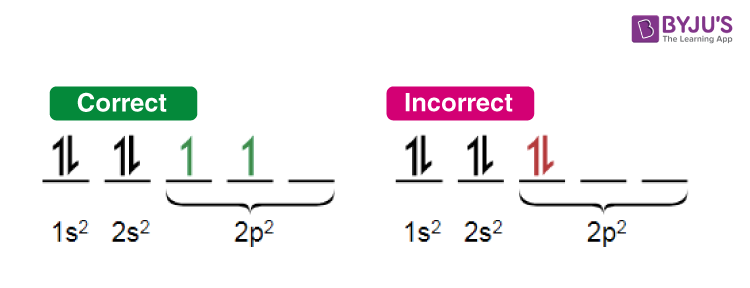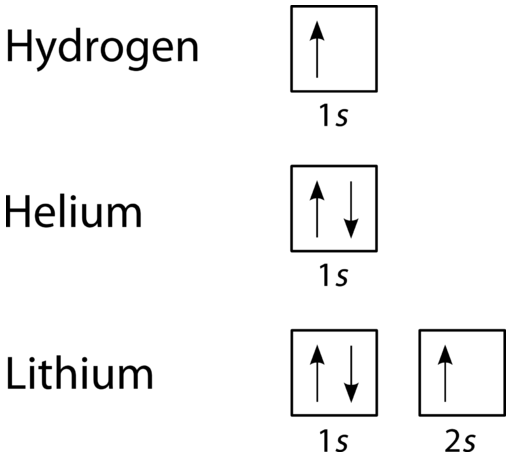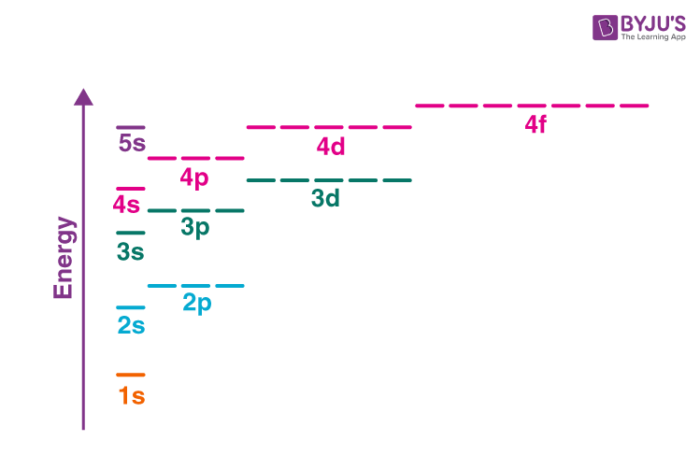Jet Set Go! All about Aeroplanes Jet Set Go! All about Aeroplanes

# Electron Configuration Questions

Electron Configuration signifies the number of electrons in an atomic orbital. The electrons are filled from the lowest energy orbital to the highest energy orbital. This representation of electron filling is given by electron configuration. The electron filled atomic orbitals are placed in a sequence. For example, Lithium (atomic number = 3) has three electrons. Its electron configuration is 1s2 2s1.

 Definition: Hund’s Rule, Pauli Exclusion Principle and Aufbau Principle are the three major rules that govern the filling of electrons in atomic subshells systematically.

## Electron Configuration Chemistry Questions with Solutions

Q1. What is the electron configuration of the element Potassium?

Answer: The atomic number of Potassium is 19. A neutral Potassium element has 19 electrons. Its electron configuration is 1s2 2s2 2p6 3s2 3p6 4s1 or simply put [Ar] 4s1.

Q2. What is the total number of electrons that can be filled in s, p and d subshells?

Answer: The s subshell can accommodate a maximum of 2 electrons as it has only 1 orbital. The p subshell has 3 orbitals. Each orbital can accommodate a maximum of 2 electrons. Thus the p subshell can accommodate a maximum of 6 electrons. The d subshell has 5 orbitals and thus can accommodate a maximum of 10 electrons.

Q3. What is Hund’s rule of maximum multiplicity? Give an example.

Answer: Hund’s rule states that the electrons singly occupy the orbitals before pairing up. Also, the singly occupied electrons in the orbitals have the same spin maximizing the total spin. This is Hund’s rule of maximum multiplicity.

Example: The correct and incorrect way of filling atomic orbitals in accordance with Hund’s rule for carbon atom (atomic number: 6) is shown below.Q4. Explain Pauli’s Exclusion Principle.

Answer: Pauli’s exclusion principle states that no two electrons in the atom can have the same set of 4 quantum numbers. The two electrons in the same orbital should have antiparallel spins.Q5. Explain Aufbau Principle.

Answer: Aufbau principle states that electrons occupy the lowest energy atomic orbitals first before occupying the higher energy atomic orbitals. The principal quantum number (n = 1, 2, 3…) signifies the energy of the orbitals. Higher the principal quantum number greater the energy of the orbital. The 1s orbital is the lowest energy orbital followed by 2s, 2p and so on.Q6. What is the maximum number of electrons that can be filled in a particular energy level?

Answer: The maximum number of electrons that can be filled in a particular energy level is 2n2, where n is the principal quantum number. For example, the maximum number of electrons in energy level n=3 is:

2 ✖ (3)2 = 18 electrons.

Q7. Match the following items of column 1 with column 2 and choose the correct answer:

Column 1 Column 2 (value of azimuthal quantum number l)
1) s orbital a) 1
2) p orbital b) 0
3) d orbital c) 2
4) f orbital d) 3

Column 1 Column 2 (value of azimuthal quantum number l)
1) s orbital b) 0
2) p orbital a) 1
3) d orbital c) 2
4) f orbital d) 3

Q8. Match the following items of column 1 with column 2 and choose the correct answer:

Column 1 Column 2
1) Carbon a) 1s2 2s2 2p3
2) Nitrogen b)1s2 2s2 2p2
3) Oxygen c)1s2 2s2 2p5
4) Fluorine d)1s2 2s2 2p4

Column 1 Column 2
1) Carbon b) 1s2 2s2 2p2
2) Nitrogen a)1s2 2s2 2p3
3) Oxygen d)1s2 2s2 2p4
4) Fluorine c)1s2 2s2 2p5

Q9. How many electrons are there in the K, L, M and N shell?

Answer: There are 2 electrons in the K shell, 8 electrons in the L shell, 18 electrons in the M shell and 32 electrons in the N shell.

Q10. Which is the orbital occupied by the last electron in the Boron element?

(a) s

(b) p

(c) d

(d) f

The electron configuration of Boron is 1s2 2s2 2p1

Q11. What is the formula to calculate azimuthal quantum number?

Answer: The azimuthal quantum number (l) is calculated by the formula l = n-1 where (n = 1,2, 3..). The value of l ranges from 0, 1, 2, 3….(n-1).

Q12. What is the formula to calculate magnetic quantum number?

Answer: The magnetic quantum number m is calculated by m = 2l + 1. The value of m ranges from -l to +l.

Q13. What are the two spin levels of electron?

Answer: The two spin levels of electron are -½ and +½.

Q14. What is the octet rule?

Answer: The octet rule states that elements attain stable electronic configuration when their valence shell is completely filled with 8 electrons.

Q15. What is the atomic number of Calcium (Ca) ?

(a) 20

(b) 19

(c) 18

(d) 17

The electronic configuration of Calcium is [Ar] 4s2.

## Practice Questions on Electronic Configuration

Q1. What is the general electronic configuration of f-block elements?

Q2. What is the general electronic configuration of Lanthanides?

Q3. What is the general electronic configuration of Actinides?

Q4. What is the electronic configuration of Neon?

Q5. What is the electronic configuration of Aluminum?

Click the PDF to check the answers for Practice Questions.

## Electron Configuration Explanation